Courses

# Power Screwdrives And Their Efficiency Mechanical Engineering Notes | EduRev

## Mechanical Engineering : Power Screwdrives And Their Efficiency Mechanical Engineering Notes | EduRev

The document Power Screwdrives And Their Efficiency Mechanical Engineering Notes | EduRev is a part of the Mechanical Engineering Course Machine Design.
All you need of Mechanical Engineering at this link: Mechanical Engineering

Introduction

A power screw is a drive used in machinery to convert a rotary motion into a linear motion for power transmission. It produces uniform motion and the design of the power screw may be such that

(a) Either the screw or the nut is held at rest and the other member rotates as it moves axially. A typical example of this is a screw clamp.

(b) Either the screw or the nut rotates but does not move axially. A typical example for this is a press. Other applications of power screws are jack screws, lead screws of a lathe, screws for vices, presses etc. Power screw normally uses square threads but ACME or Buttress threads may also be used. Power screws should be designed for smooth and noiseless transmission of power with an ability to carry heavy loads with high efficiency. We first consider the different thread forms and their proportions:

Square threads

The thread form is shown in figure-6.1.1.1. These threads have high efficiency but they are difficult to manufacture and are expensive. The proportions in terms of pitch are: h1= 0.5 p ; h2 = 0.5 p - b ; H = 0.5 p + a ; e = 0.5 p a and b are different for different series of threads.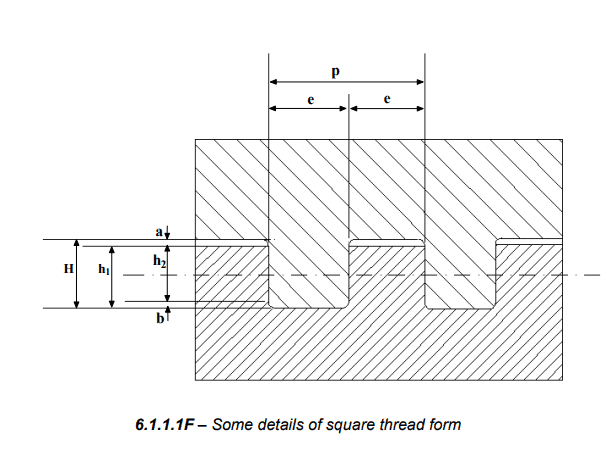There are different series of this thread form and some nominal diameters, corresponding pitch and dimensions a and b are shown in table-6.1.1.1 as per I.S. 4694-1968.

6.1.1.1T – Dimensions of three different series of square thread form.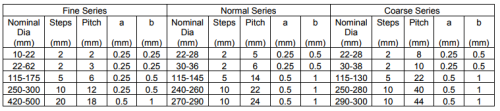According to IS-4694-1968, a square thread is designated by its nominal diameter and pitch, as for example, SQ 10 x 2 designates a thread form of nominal diameter 10 mm and pitch 2 mm.

Acme or Trapezoidal threads

The Acme thread form is shown in figure- 6.1.1.2. These threads may be used in applications such as lead screw of a lathe where loss of motion cannot be tolerated. The included angle 2φ = 29o and other proportions are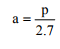and h = 0.25 p + 0.25 mm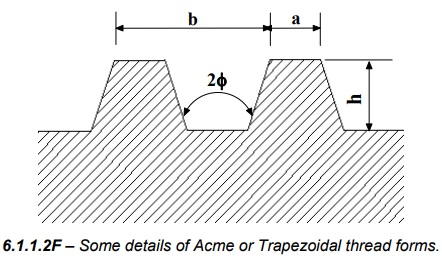A metric trapezoidal thread form is shown in figure- 6.1.1.3 and different proportions of the thread form in terms of the pitch are as follows: Included angle = 30o ; H1= 0.5 p ; z = 0.25 p + H1/2 ; H3 = h3 = H1+ ac = 0.5 p + ac

ac is different for different pitch, for example
ac = 0.15 mm for p = 1.5 mm ; ac = 0.25 mm for p = 2 to 5 mm;
a = 0.5 mm for p = 6 to 12 mm ; a= 1 mm for p = 14 to 44 mm.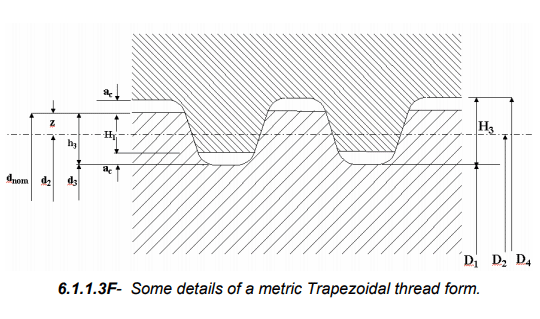Some standard dimensions for a trapezoidal thread form are given in table- 6.1.1.2 as per IS 7008 (Part II and III) - 1973: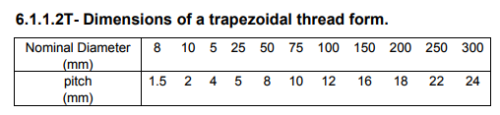According to IS7008-1973 trapezoidal threads may be designated as, for example, Tr 50 x 8 which indicates a nominal diameter of 50 mm and a pitch of 8 mm.

Buttress thread

This thread form can also be used for power screws but they can transmit power only in one direction. Typical applications are screw jack, vices etc. A Buttress thread form is shown in figure- 6.1.1.4. and the proportions are shown in the figure in terms of the pitch. On the whole the square threads have the highest efficiency as compared to other thread forms but they are less sturdy than the trapezoidal thread forms and the adjustment for wear is difficult for square threads.

When a large linear motion of a power screw is required two or more parallel threads are used. These are called multiple start power drives.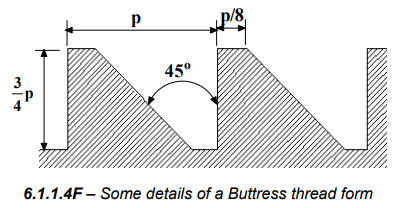Efficiency of a power screw

A square thread power screw with a single start is shown in figure6.1.2.1. Here p is the pitch, α the helix angle, dm the mean diameter of thread and F is the axial load. A developed single thread is shown in figure- 6.1.2.2 where L = n p for a multi-start drive, n being the number of starts. In order to analyze the mechanics of the power screw we need to consider two cases:

(a) Raising the load
(b) Lowering the load.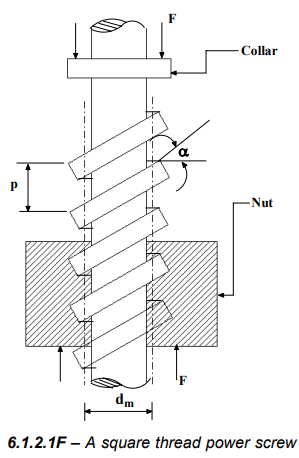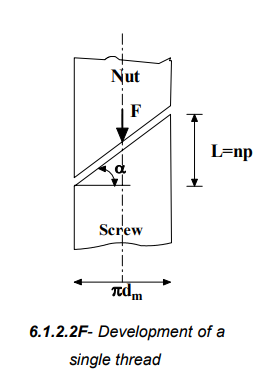Raising the load This requires an axial force P as shown in figure- 6.1.2.3. Here N is the normal reaction and μN is the frictional force. For equilibrium

P - μ N cos α - N sin α = 0
F + μ N sin α - N cos α = 0

This gives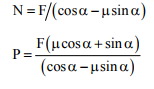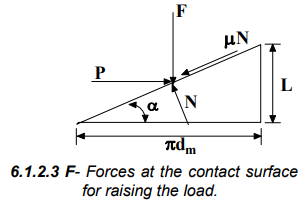Torque transmitted during raising the load is then given by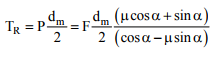Since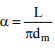we have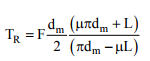The force system at the thread during lowering the load is shown in figure- 6.1.2.4. For equilibrium

P - μ N cos α + N sin α = 0
F - N cos α -μ N sin α = 0

This gives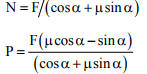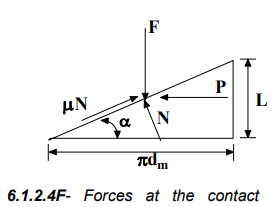Torque required to lower the load is given by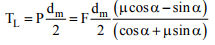And again taking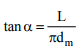we have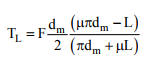Condition for self locking

The load would lower itself without any external force if μπdm < L and some external force is required to lower the load if μπdm < L

This is therefore the condition for self locking.

Efficiency of the power screw is given by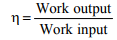Here work output = F. L

Work input = p. πdm

This gives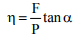The above analysis is for square thread and for trapezoidal thread some modification is required. Because of the thread angle the force normal to the thread surface is increased as shown in figure- 6.1.2.5. The torque is therefore given by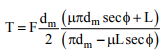This considers the increased friction due to the wedging action. The trapezoidal threads are not preferred because of high friction but often used due to their ease of machining.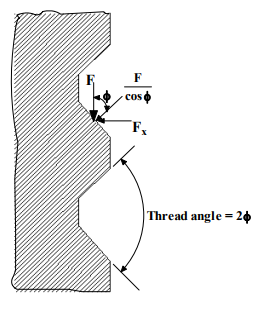Bursting effect on the nut

Bursting effect on the nut is caused by the horizontal component of the axial load F on the screw and this is given by ( figure- 6.1.2.5)

F= F tan φ

For an ISO metric nut 2φ = 60o and Fx = 0.5777 F.

Collar friction

If collar friction μc is considered then another term μFdc/2 must be added to torque expression. Here dc is the effective friction diameter of the collar. Therefore we may write the torque required to raise the load as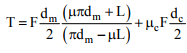Offer running on EduRev: Apply code STAYHOME200 to get INR 200 off on our premium plan EduRev Infinity!

## Machine Design

57 videos|71 docs|102 tests

,

,

,

,

,

,

,

,

,

,

,

,

,

,

,

,

,

,

,

,

,

;